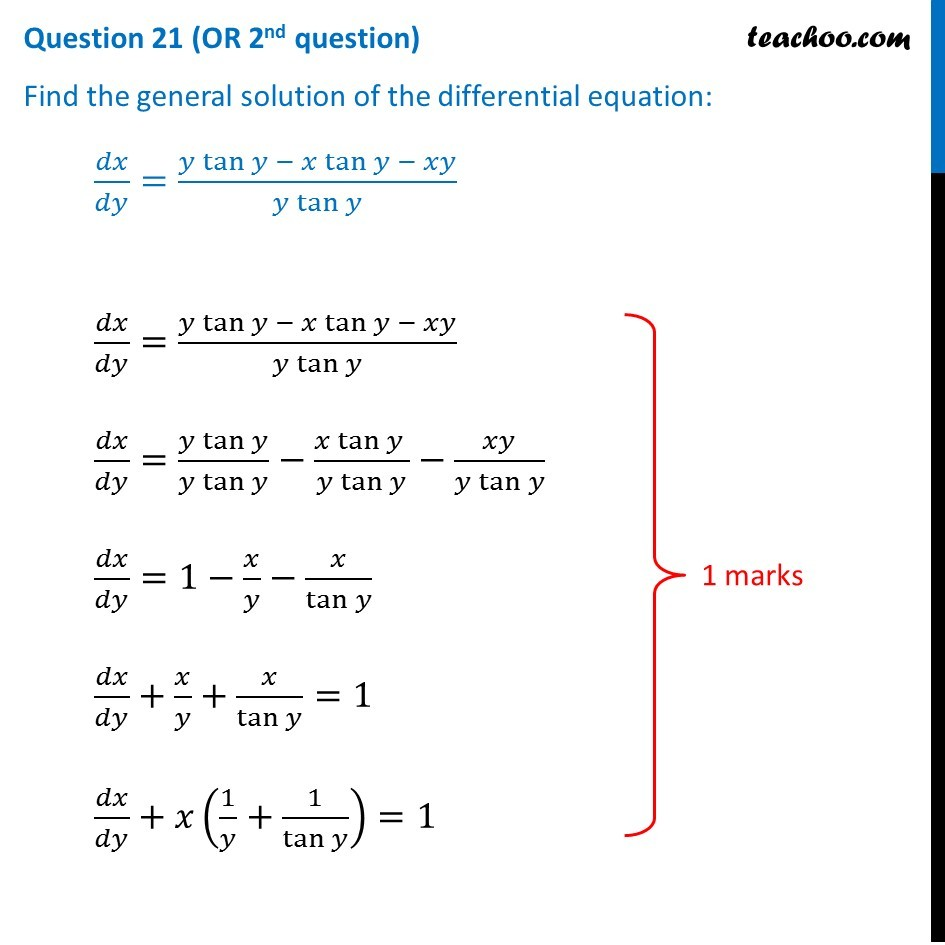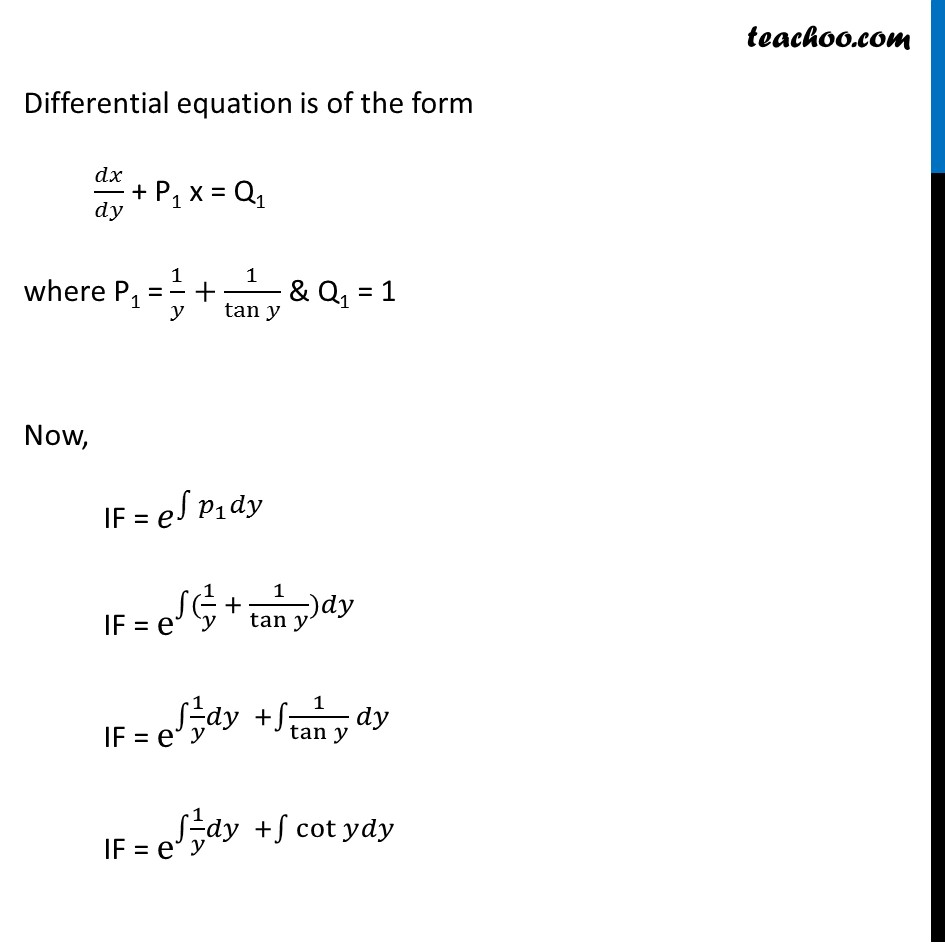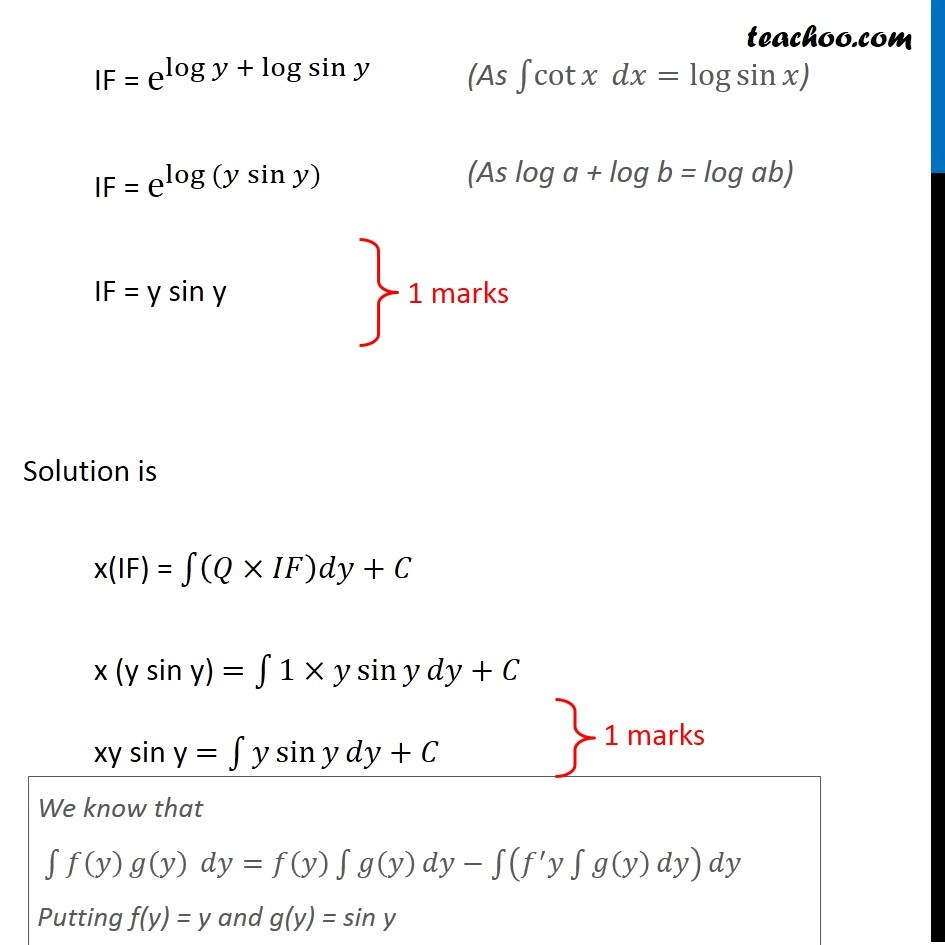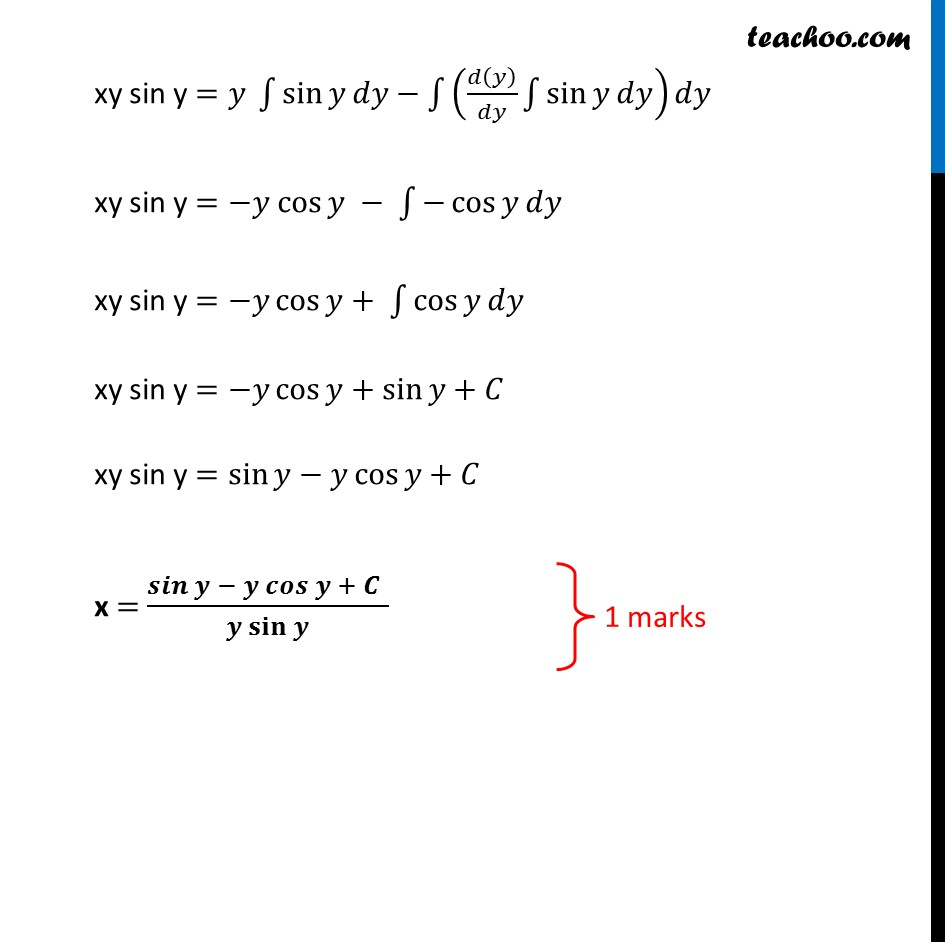CBSE Class 12 Sample Paper for 2019 Boards

Class 12
Solutions of Sample Papers and Past Year Papers - for Class 12 Boards

Question 21 (OR 2 nd question)

Find the general solution of the differential equation:

dx/dy = (y tan⁡y  - x tan⁡y  - xy) / (y tan⁡y)Get live Maths 1-on-1 Classs - Class 6 to 12

### Transcript

Question 21 (OR 2nd question) Find the general solution of the differential equation: 𝑑𝑥/𝑑𝑦=(𝑦 tan⁡𝑦 − 𝑥 tan⁡𝑦 − 𝑥𝑦)/(𝑦 tan⁡𝑦 ) 𝑑𝑥/𝑑𝑦=(𝑦 tan⁡𝑦 − 𝑥 tan⁡𝑦 − 𝑥𝑦)/(𝑦 tan⁡𝑦 ) 𝑑𝑥/𝑑𝑦=(𝑦 tan⁡𝑦)/(𝑦 tan⁡𝑦 )−(𝑥 tan⁡𝑦 )/(𝑦 tan⁡𝑦 )−𝑥𝑦/(𝑦 tan⁡𝑦 ) 𝑑𝑥/𝑑𝑦=1−𝑥/𝑦−𝑥/tan⁡𝑦 𝑑𝑥/𝑑𝑦+𝑥/𝑦+𝑥/tan⁡𝑦 =1 𝑑𝑥/𝑑𝑦+𝑥(1/𝑦+1/tan⁡𝑦 )=1 Differential equation is of the form 𝑑𝑥/𝑑𝑦 + P1 x = Q1 where P1 = 1/𝑦+1/tan⁡𝑦 & Q1 = 1 Now, IF = 𝑒^∫1▒〖𝑝_1 𝑑𝑦〗 IF = e^∫1▒〖(1/𝑦 + 1/tan⁡𝑦 )𝑑𝑦" " 〗 IF = e^(∫1▒〖1/𝑦 𝑑𝑦〗 +∫1▒〖1/tan⁡𝑦 𝑑𝑦〗) IF = e^(∫1▒〖1/𝑦 𝑑𝑦〗 +∫1▒〖cot⁡𝑦 𝑑𝑦〗) IF = e^(log⁡𝑦 + log⁡sin⁡𝑦 ) IF = e^〖log 〗⁡〖(𝑦 sin⁡𝑦)〗 IF = y sin y Solution is x(IF) = ∫1▒〖(𝑄×𝐼𝐹)𝑑𝑦+𝐶 〗 x (y sin y) =∫1▒〖1×𝑦 sin⁡𝑦 〗 𝑑𝑦+𝐶 xy sin y =∫1▒〖𝑦 sin⁡𝑦 〗 𝑑𝑦+𝐶 (As ∫1▒cot⁡𝑥 𝑑𝑥=log⁡sin⁡𝑥 ) (As log a + log b = log ab) We know that ∫1▒〖𝑓(𝑦) 𝑔⁡(𝑦) 〗 𝑑𝑦=𝑓(𝑦) ∫1▒𝑔(𝑦) 𝑑𝑦−∫1▒(𝑓^′ 𝑦∫1▒𝑔(𝑦) 𝑑𝑦) 𝑑𝑦 Putting f(y) = y and g(y) = sin y xy sin y =𝑦" " ∫1▒sin⁡𝑦 𝑑𝑦−∫1▒(𝑑(𝑦)/𝑑𝑦 ∫1▒〖sin⁡𝑦 𝑑𝑦〗) 𝑑𝑦 xy sin y =−𝑦 cos⁡𝑦 − ∫1▒〖−cos⁡𝑦 𝑑𝑦〗 xy sin y =−𝑦 cos⁡𝑦+ ∫1▒〖cos⁡𝑦 𝑑𝑦〗 xy sin y =−𝑦 cos⁡𝑦+sin⁡𝑦+𝐶 xy sin y =sin⁡𝑦−𝑦 cos⁡𝑦+𝐶 x = (𝒔𝒊𝒏⁡𝒚 − 𝒚 𝒄𝒐𝒔⁡𝒚 + 𝑪" " )/(𝒚 𝐬𝐢𝐧⁡𝒚 )